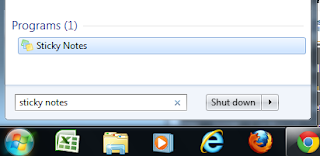# Windows 7 Sticky Notes Tips

By | February 20, 2013Sticky Note Short Cuts

CTRL + L = Left Alignment

CTRL + E = Center Alignment

CTRL + R = Right Alignment

CTRL + B = Bold

CTRL + I = Italic

CTRL + U = Underline

CTRL + T = Strike Through

CTRL + SHIFT + > = Increase Font Size

CTRL + SHIFT + < = Decrease Font Size

CTRL + SHIFT + L (1 Time) = Bullet List (●, ●, ●)

CTRL + SHIFT + L (2 Times) = Numbered List (1, 2, 3)

CTRL + SHIFT + L (3 Times) = Lettered List (a, b, c)

CTRL + SHIFT + L (4 Times) = Lettered List (A, B, C)

CTRL + SHIFT + L (5 Times) = Roman Numeral List (i, ii, iii)

CTRL + SHIFT + L (6 Times) = Roman Numeral List (I, II, III)

CTRL + N = New Note

CTRL + D = Delete Note

CTRL + A = Select All

CTRL + X = Cut

CTRL + C = Copy

CTRL + V = Paste

CTRL + Z = Undo

CTRL + Y = Red

.